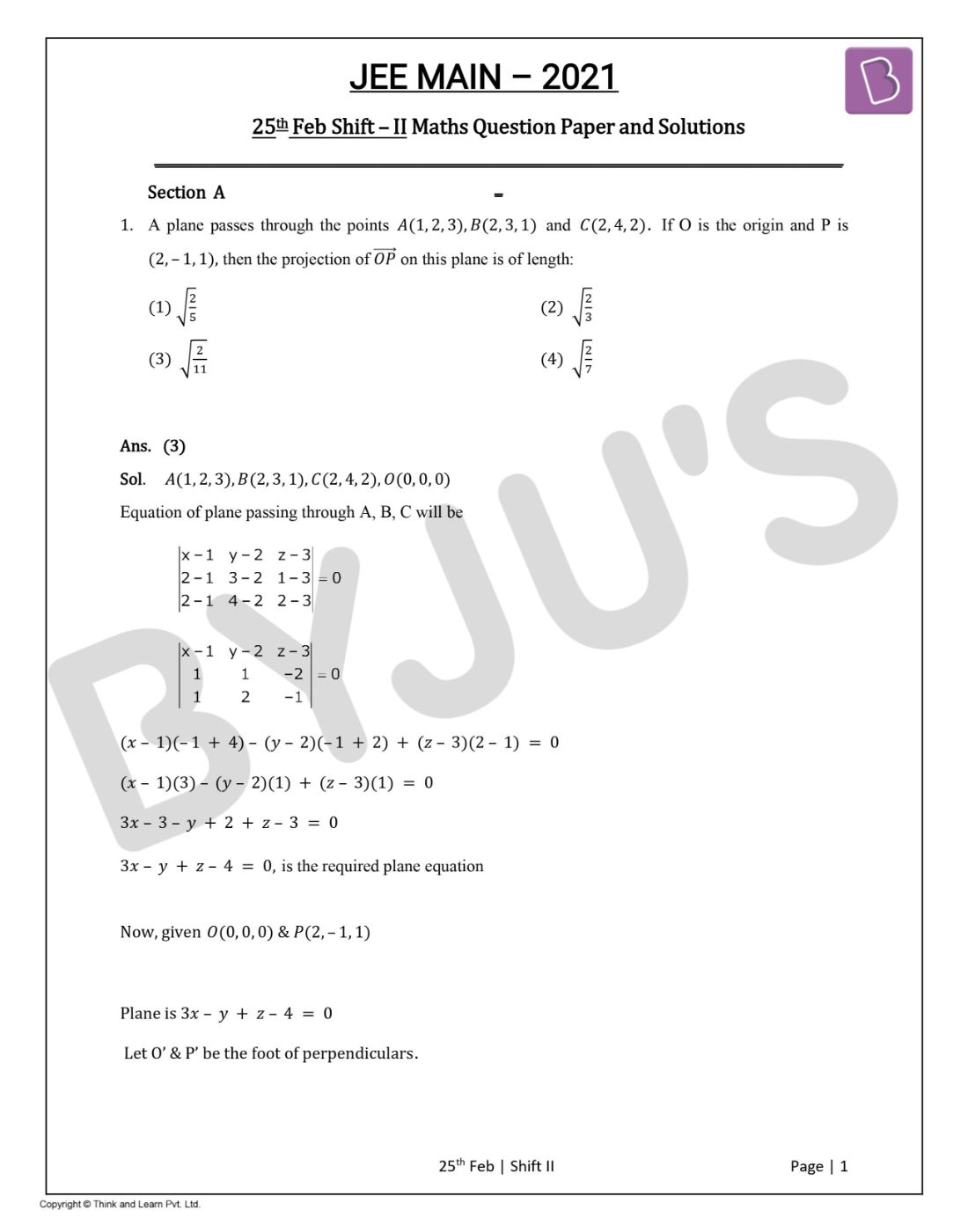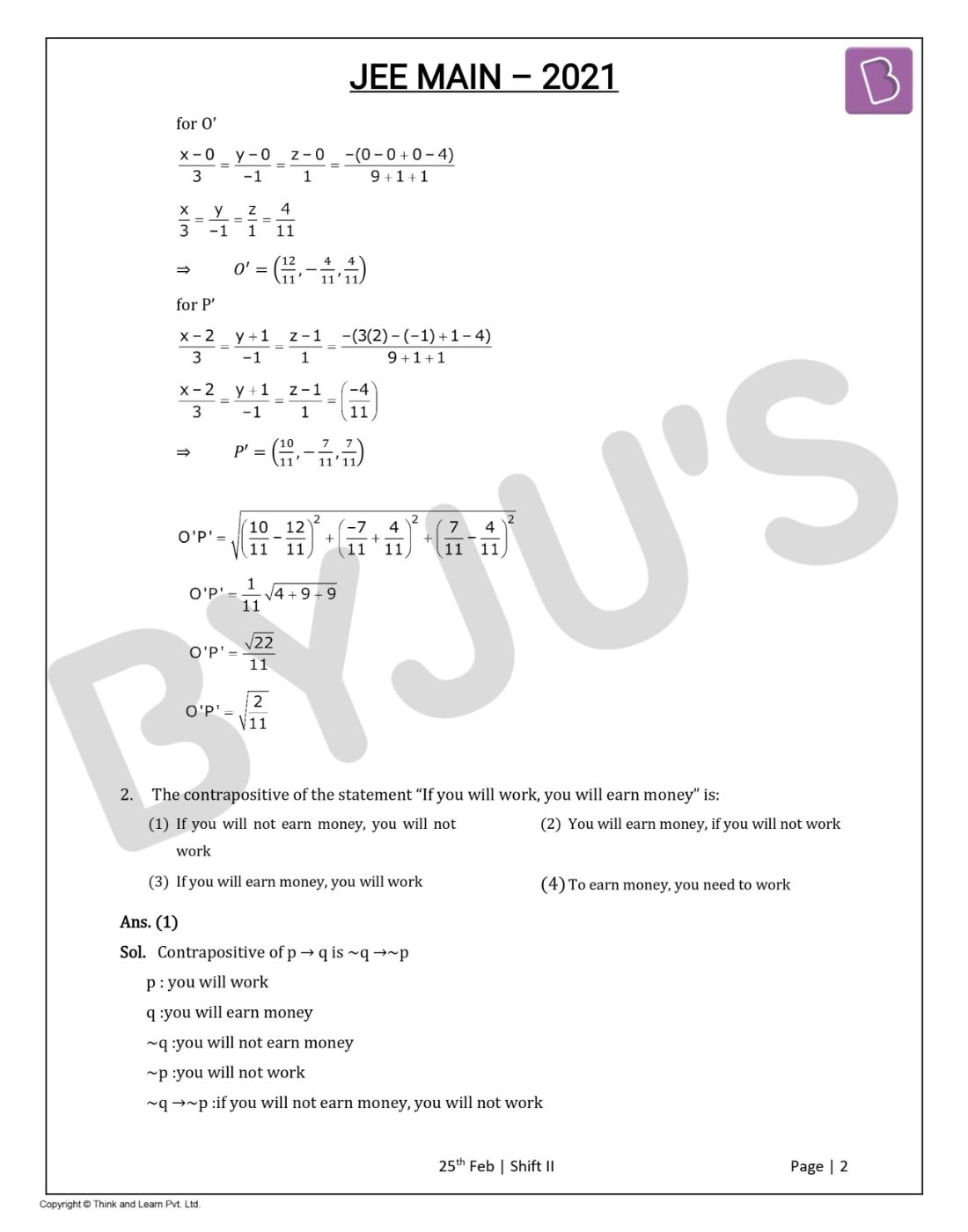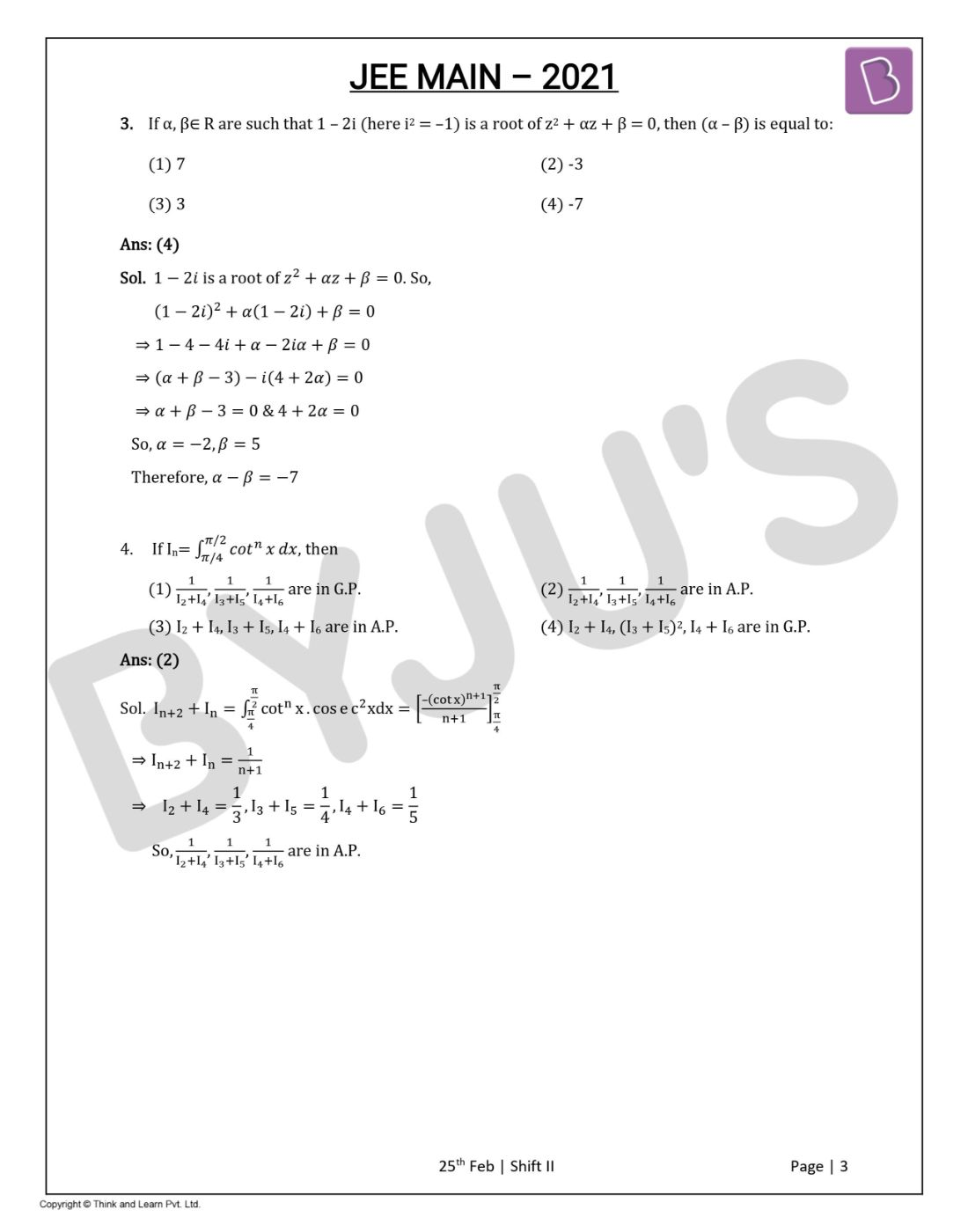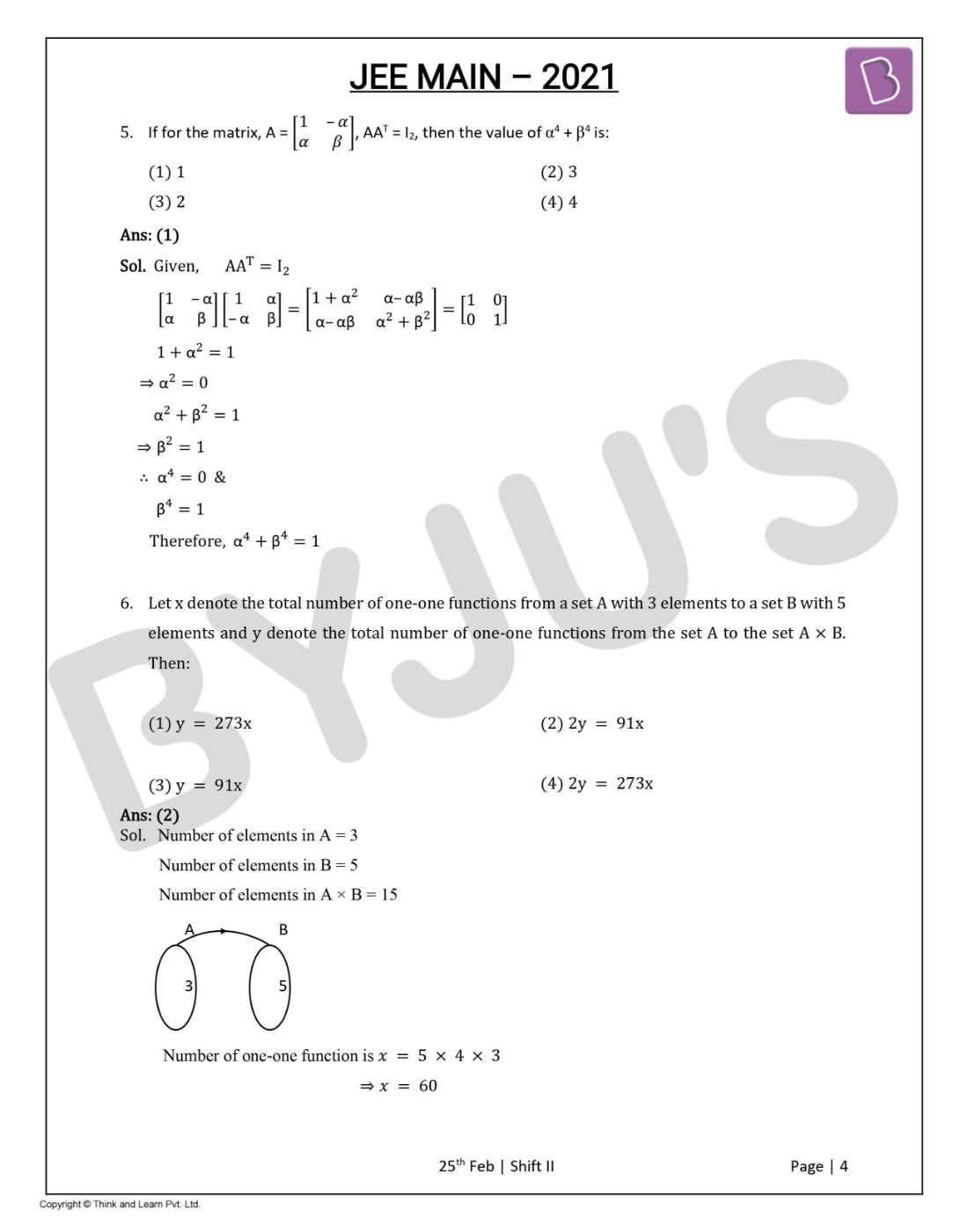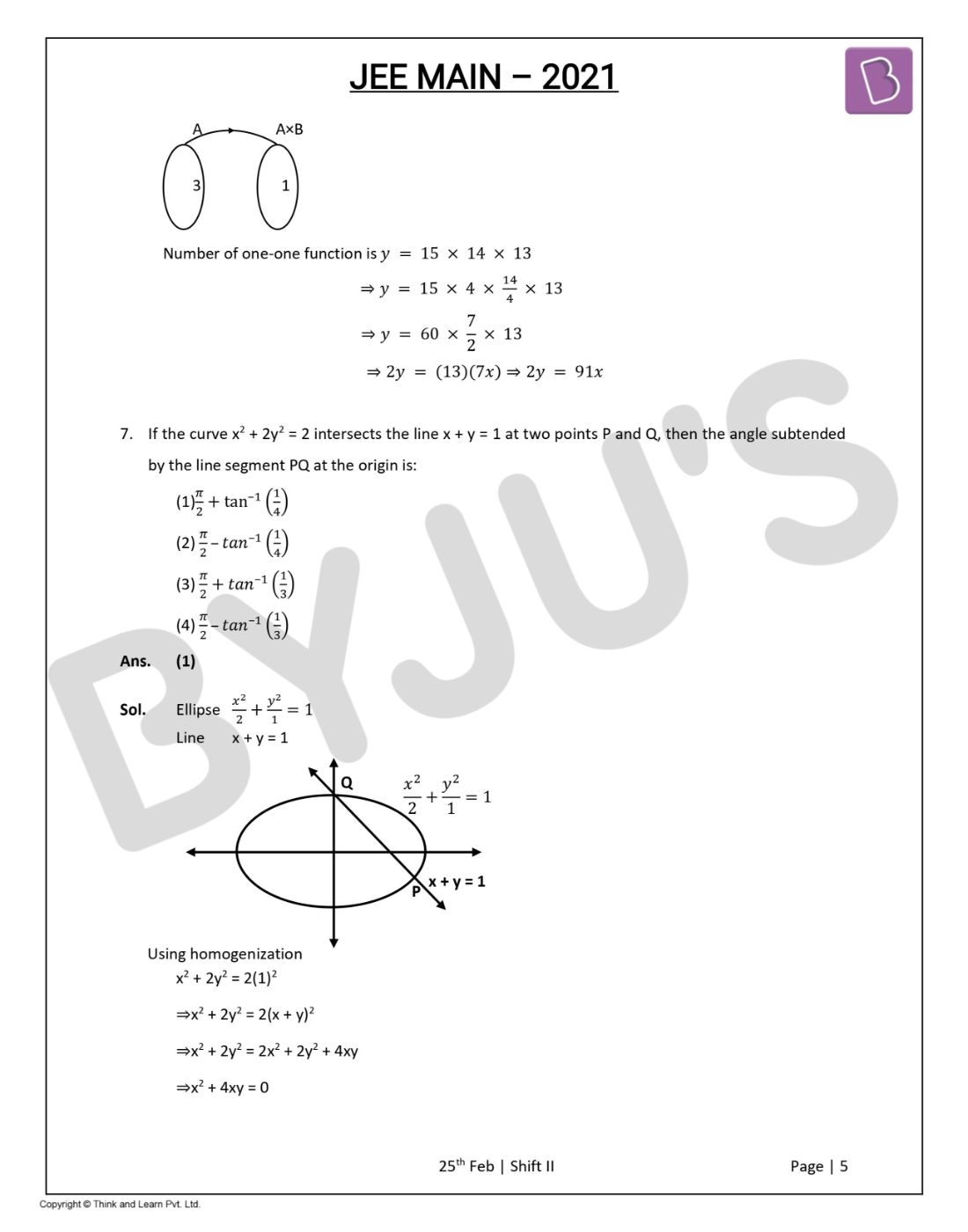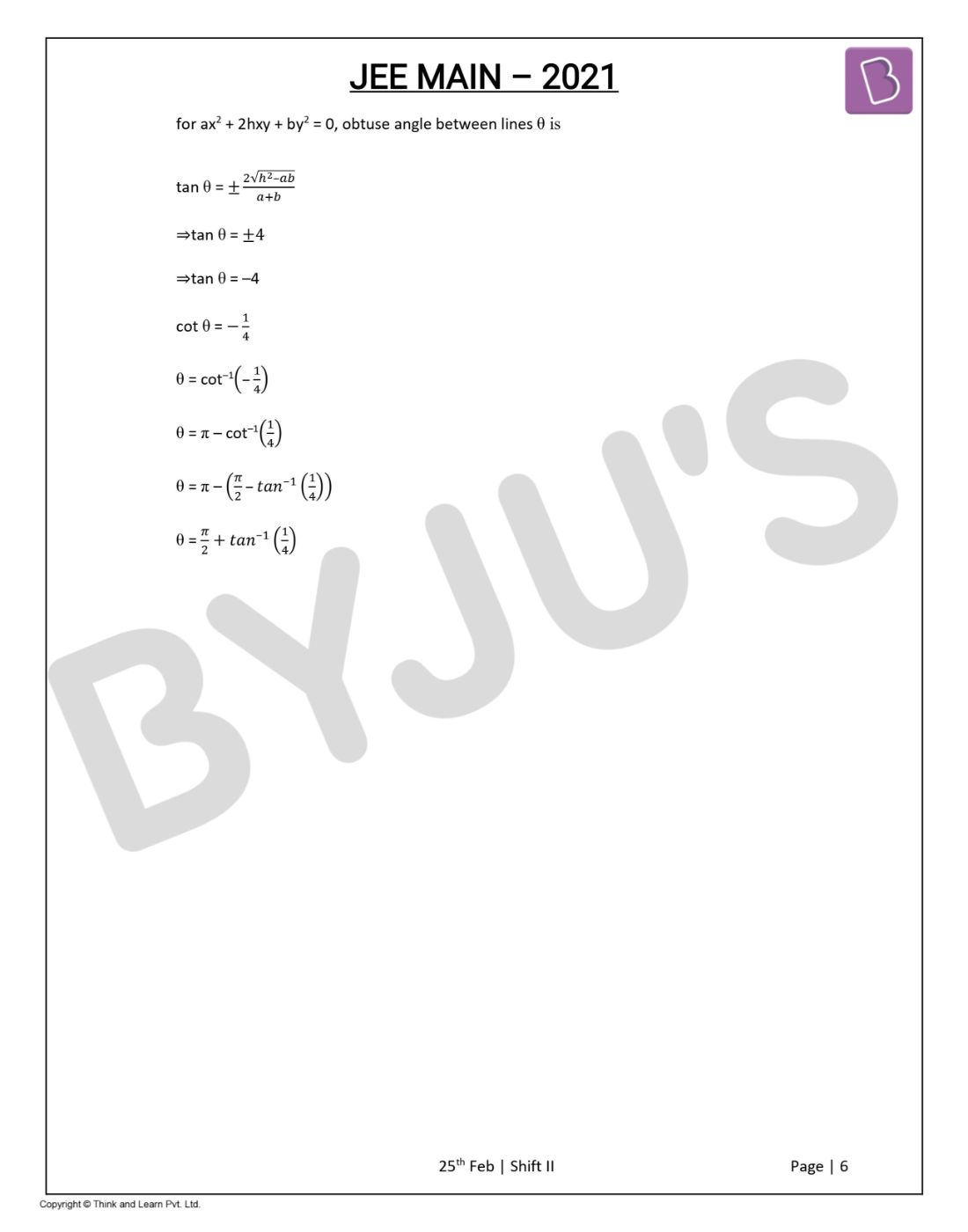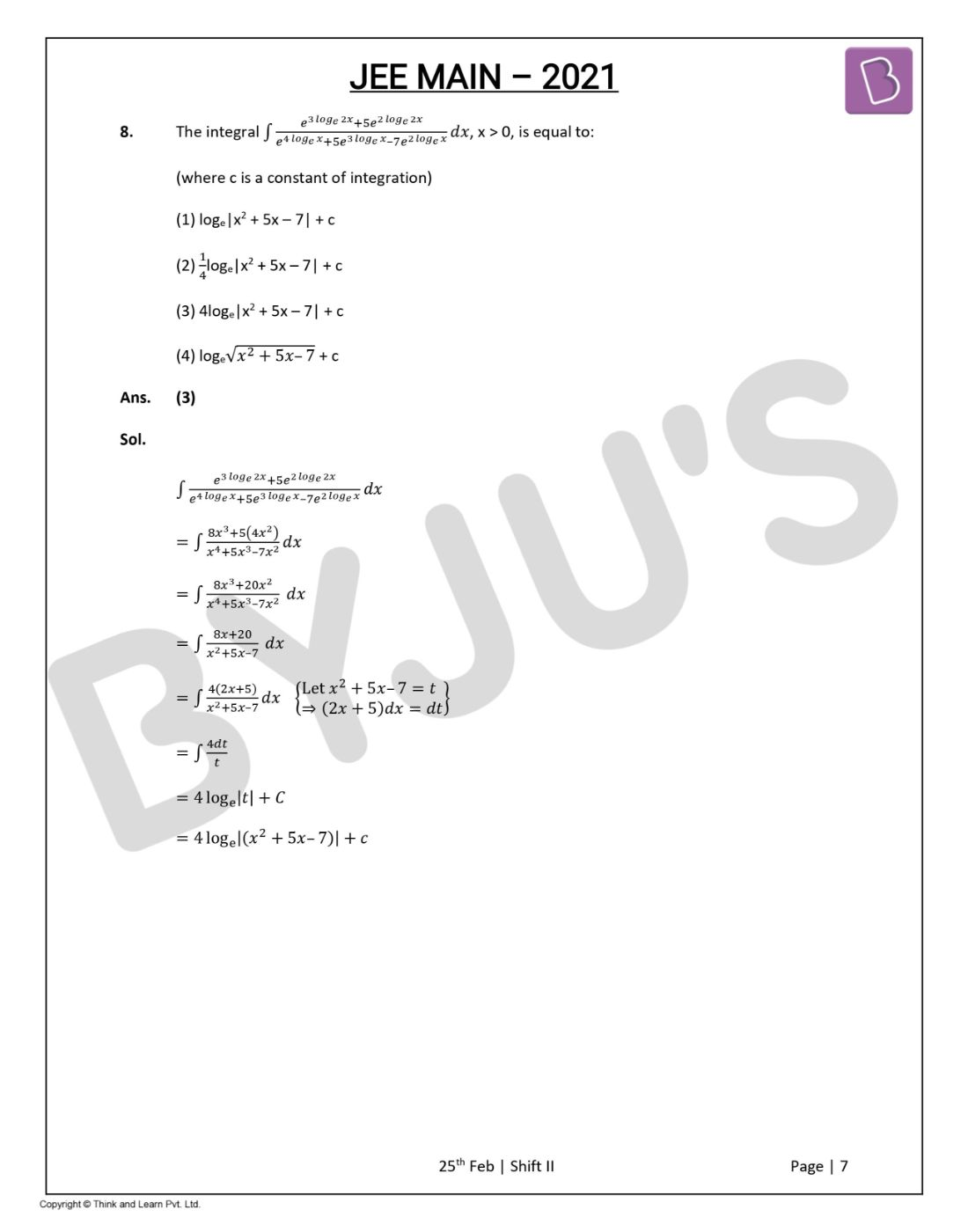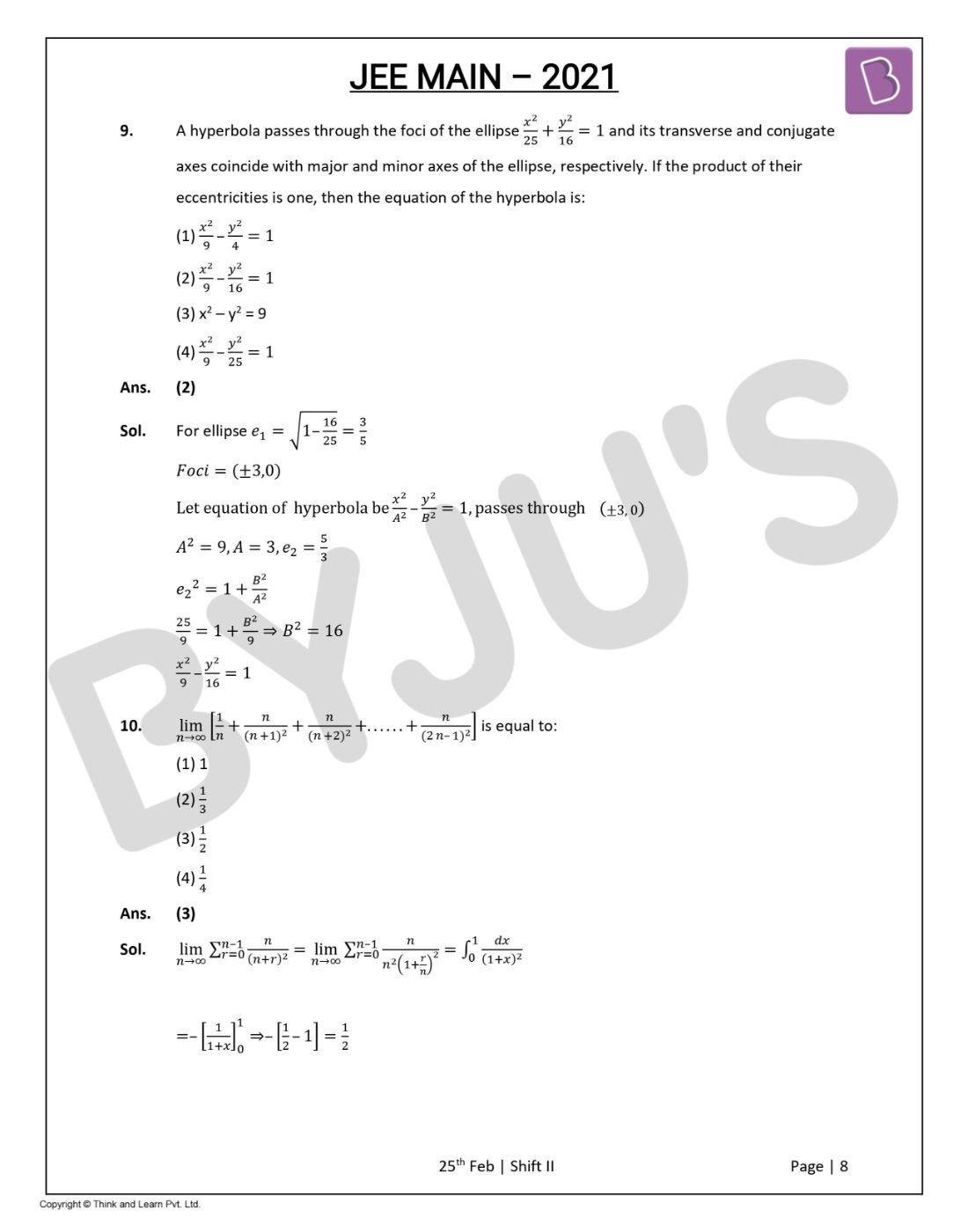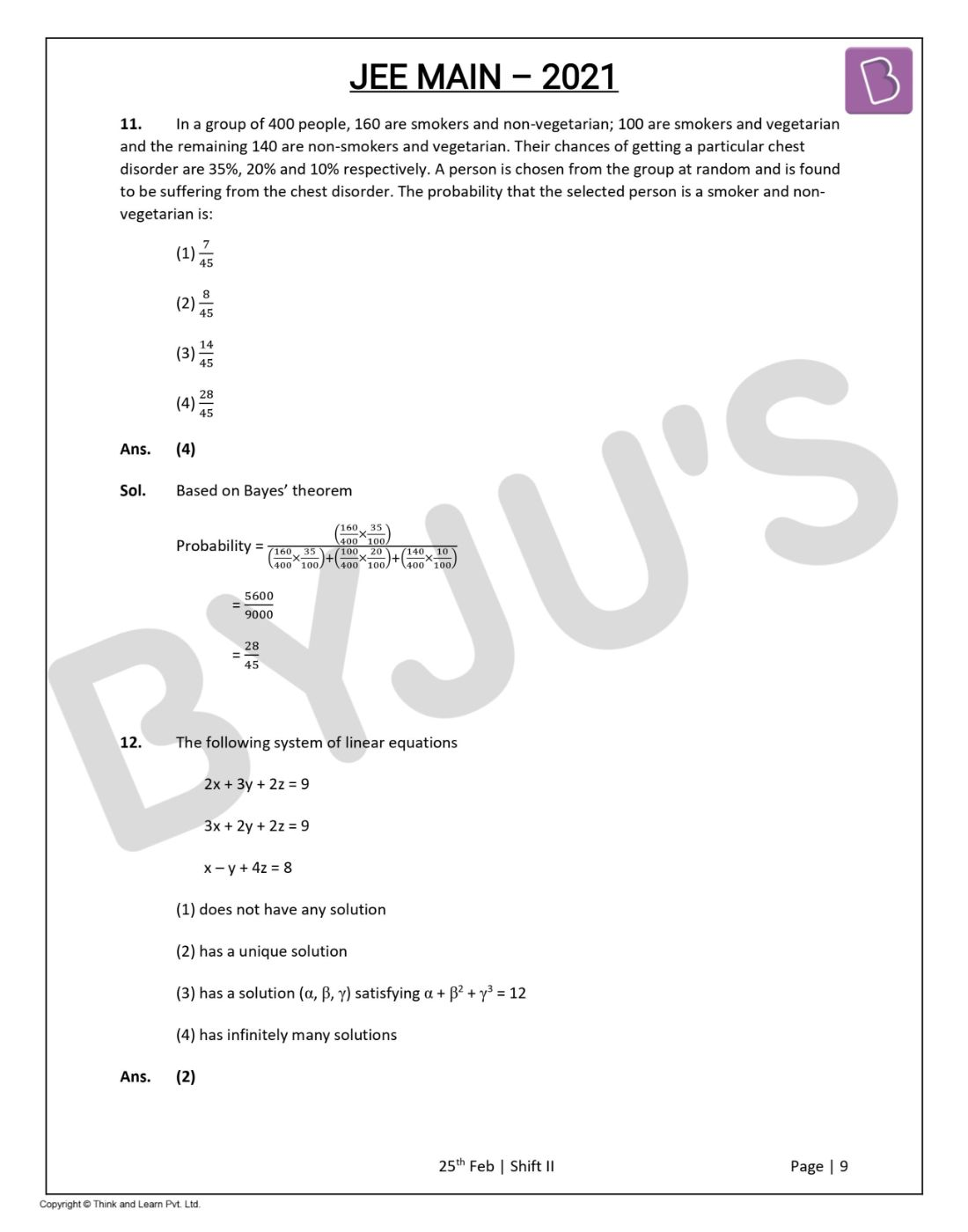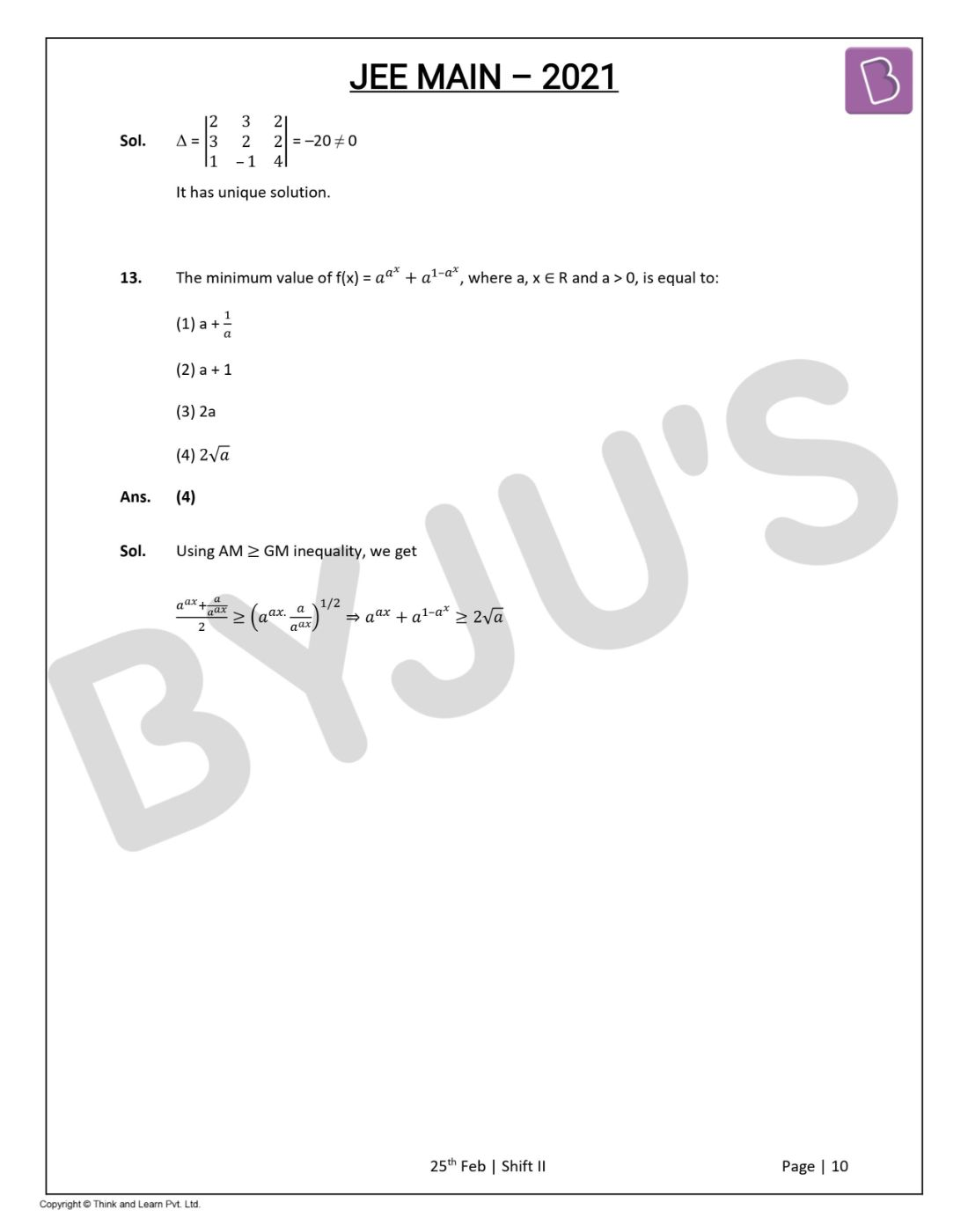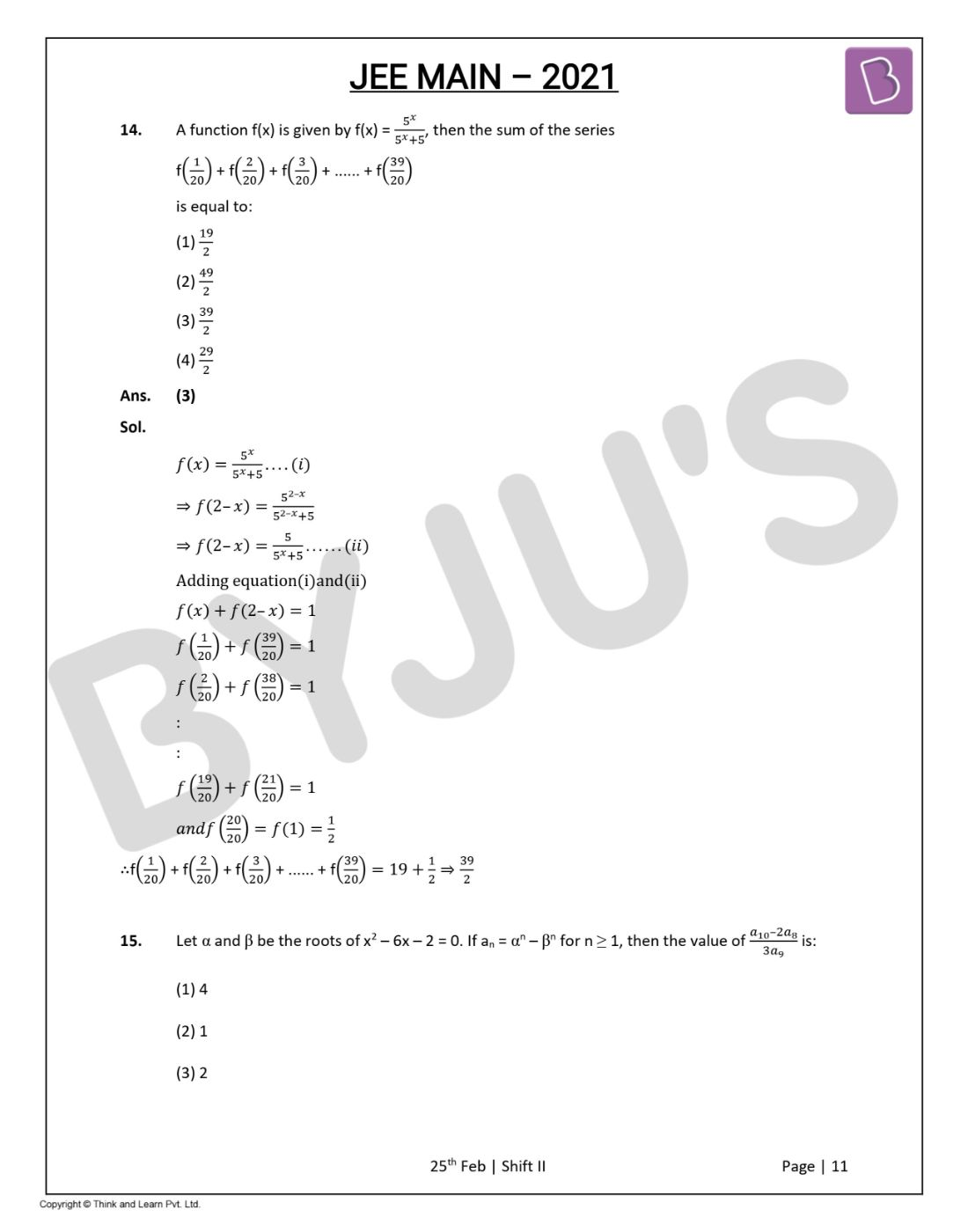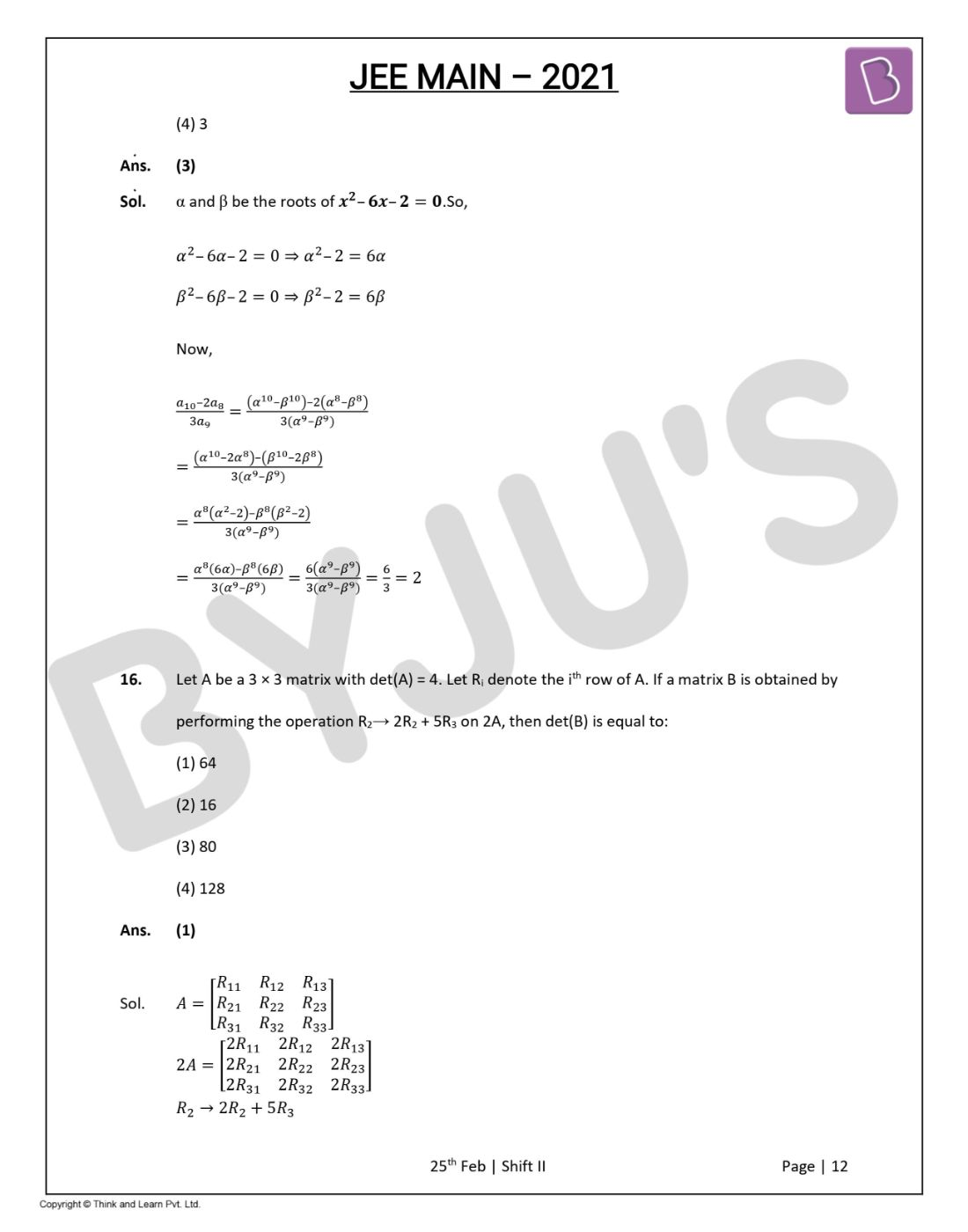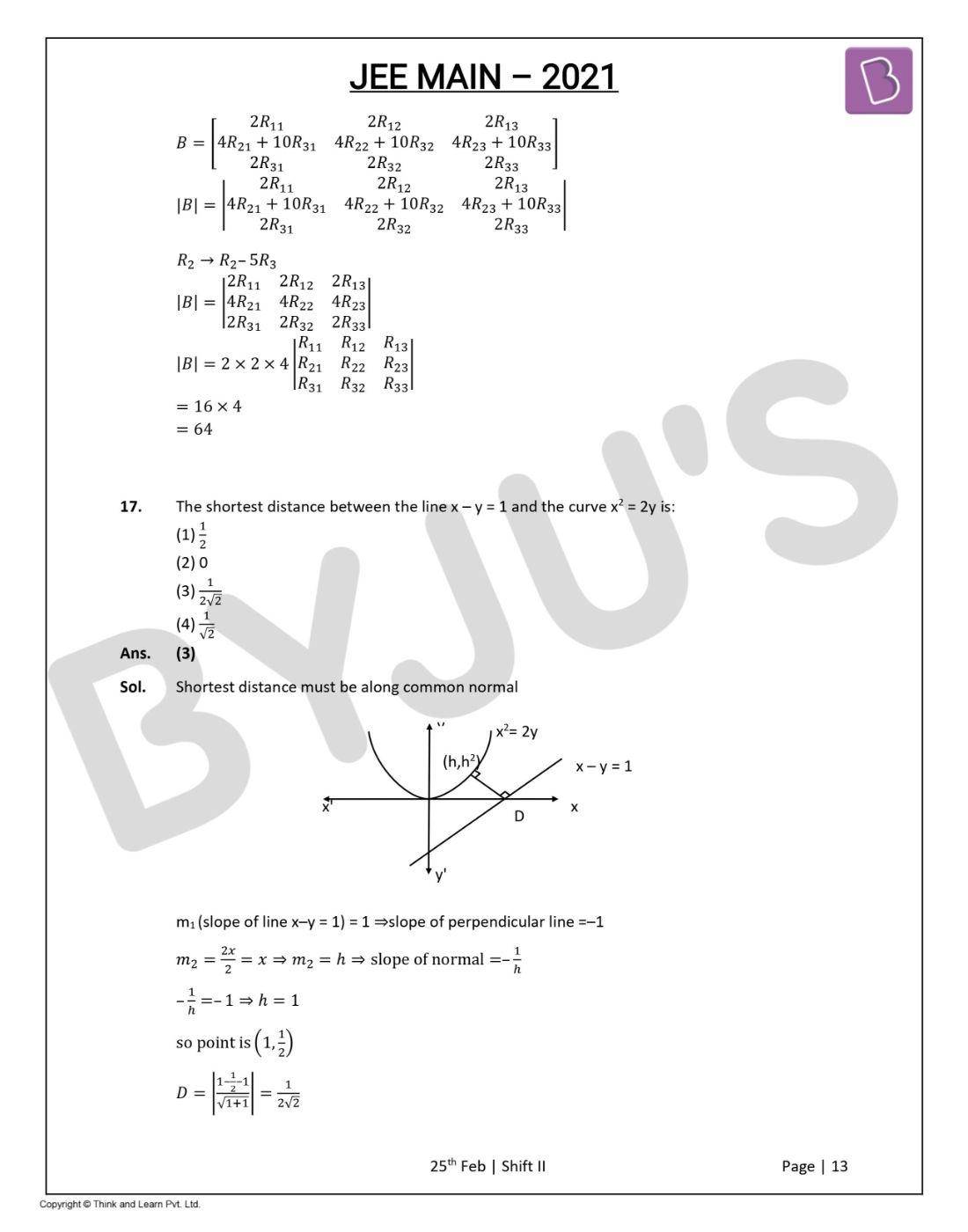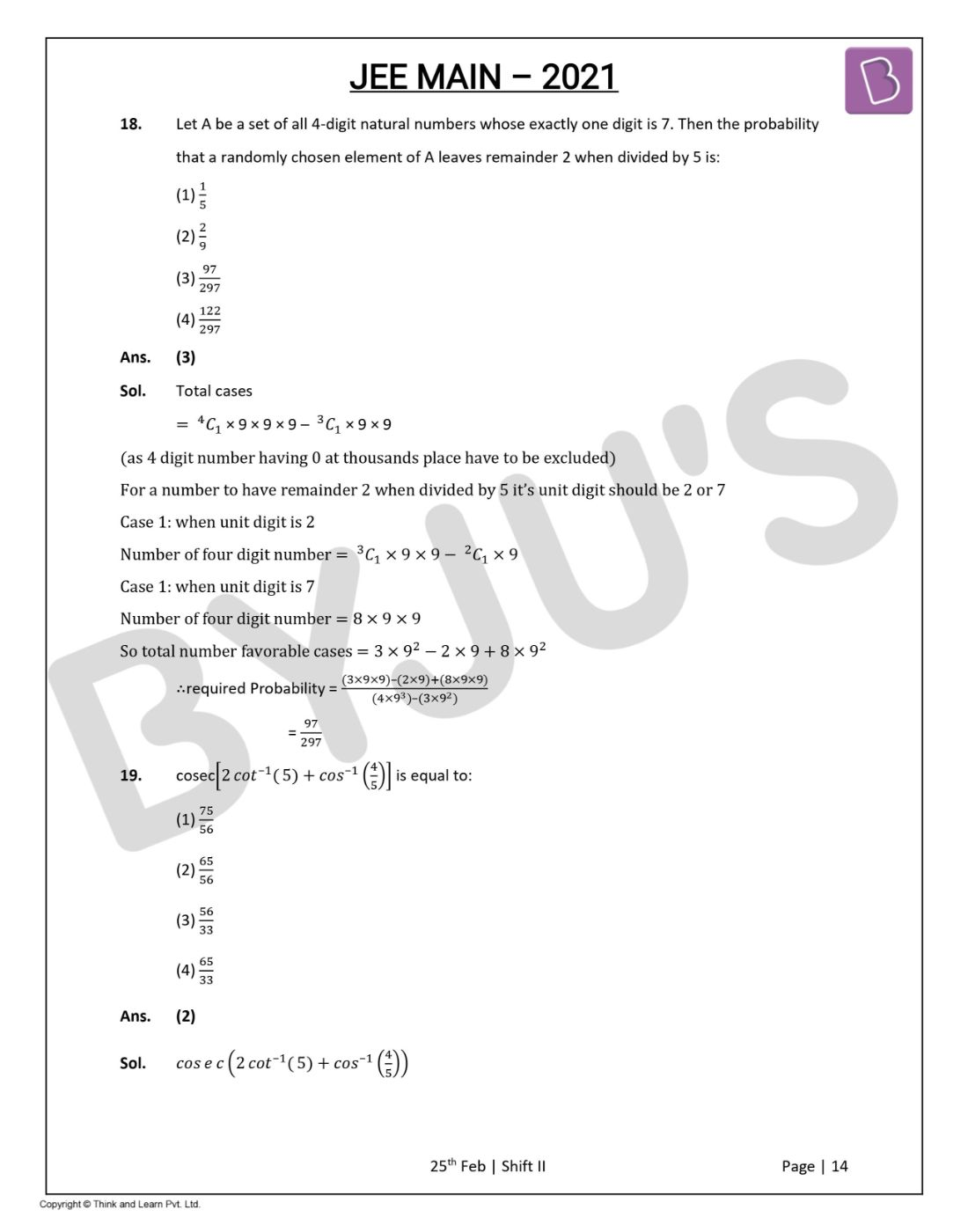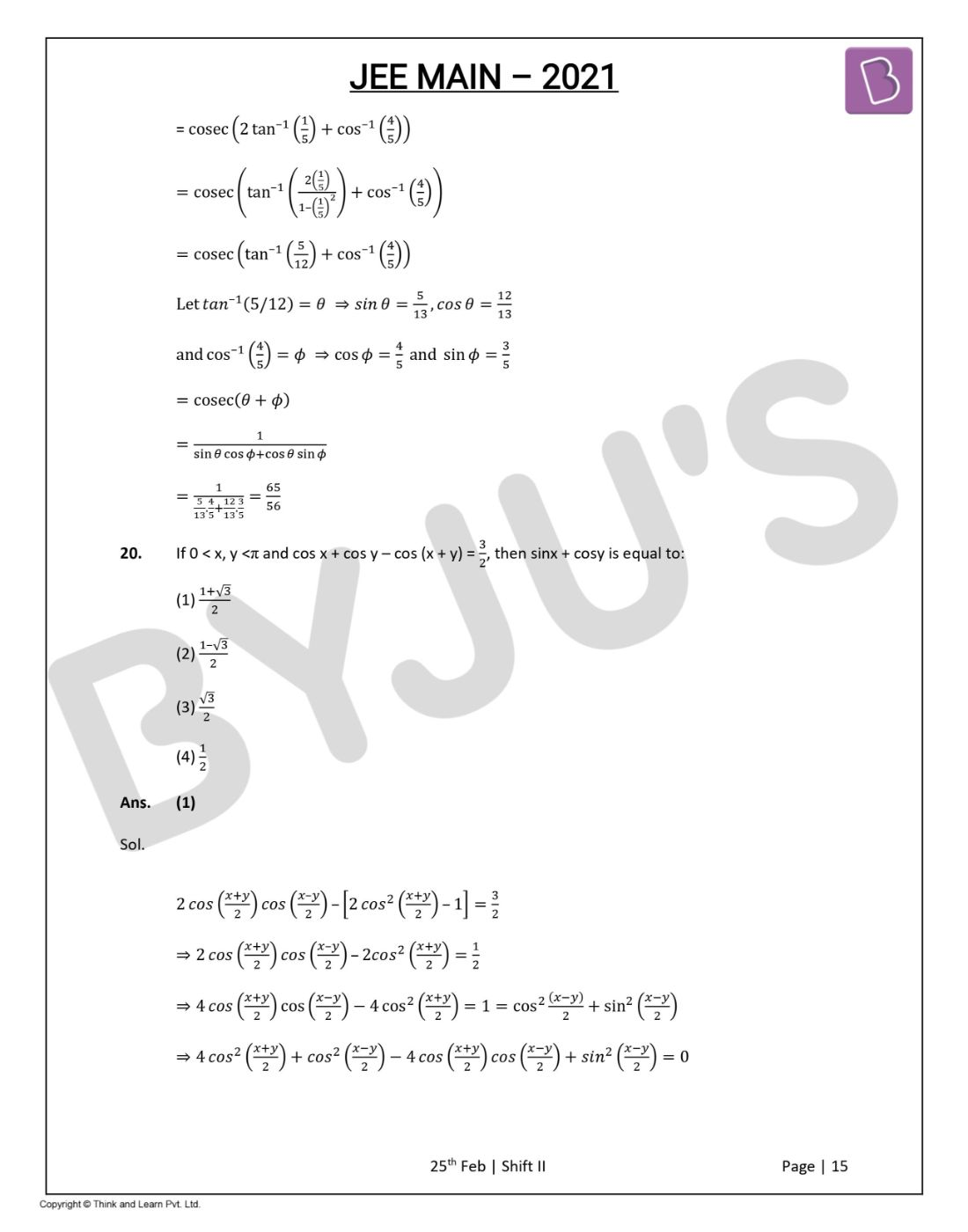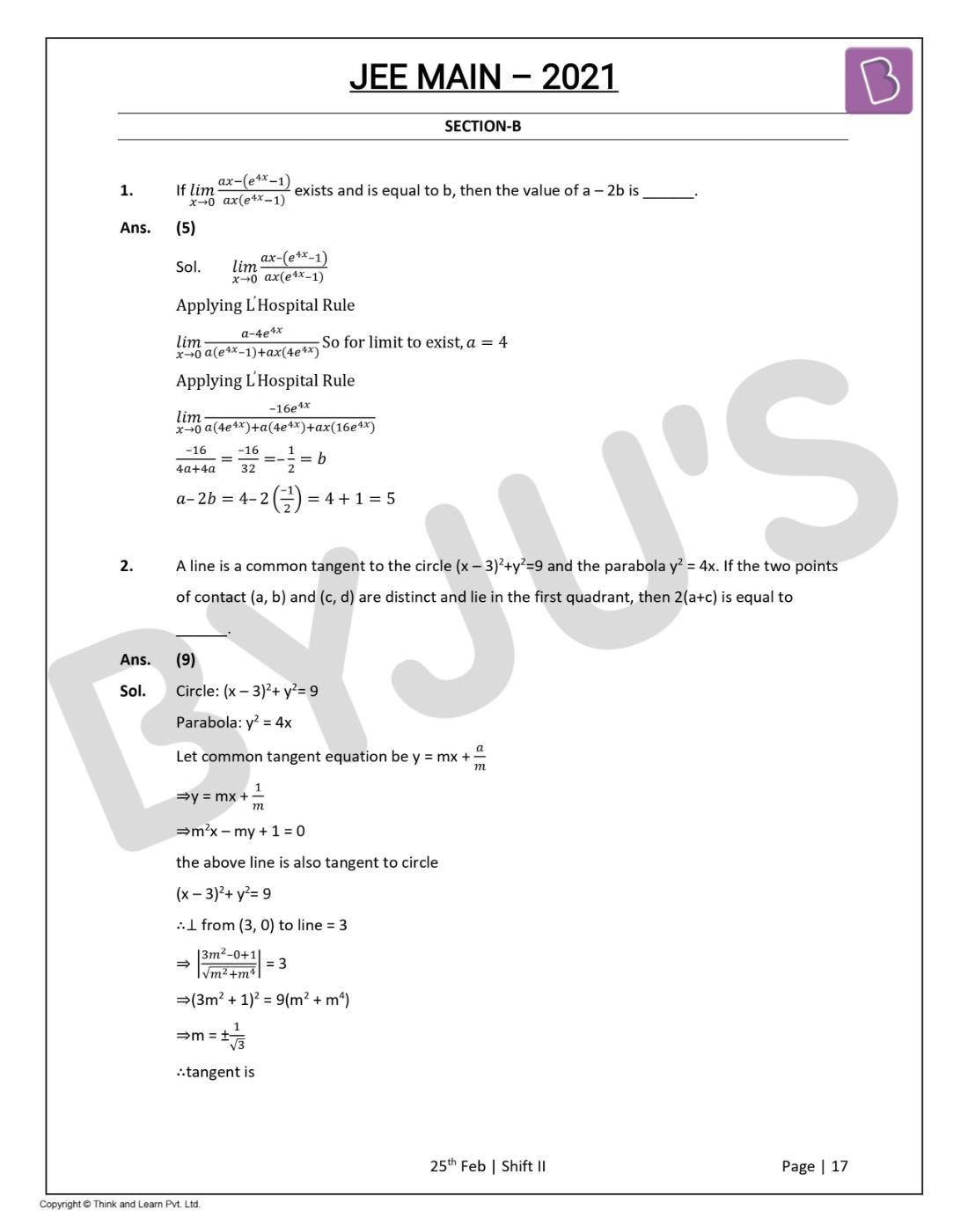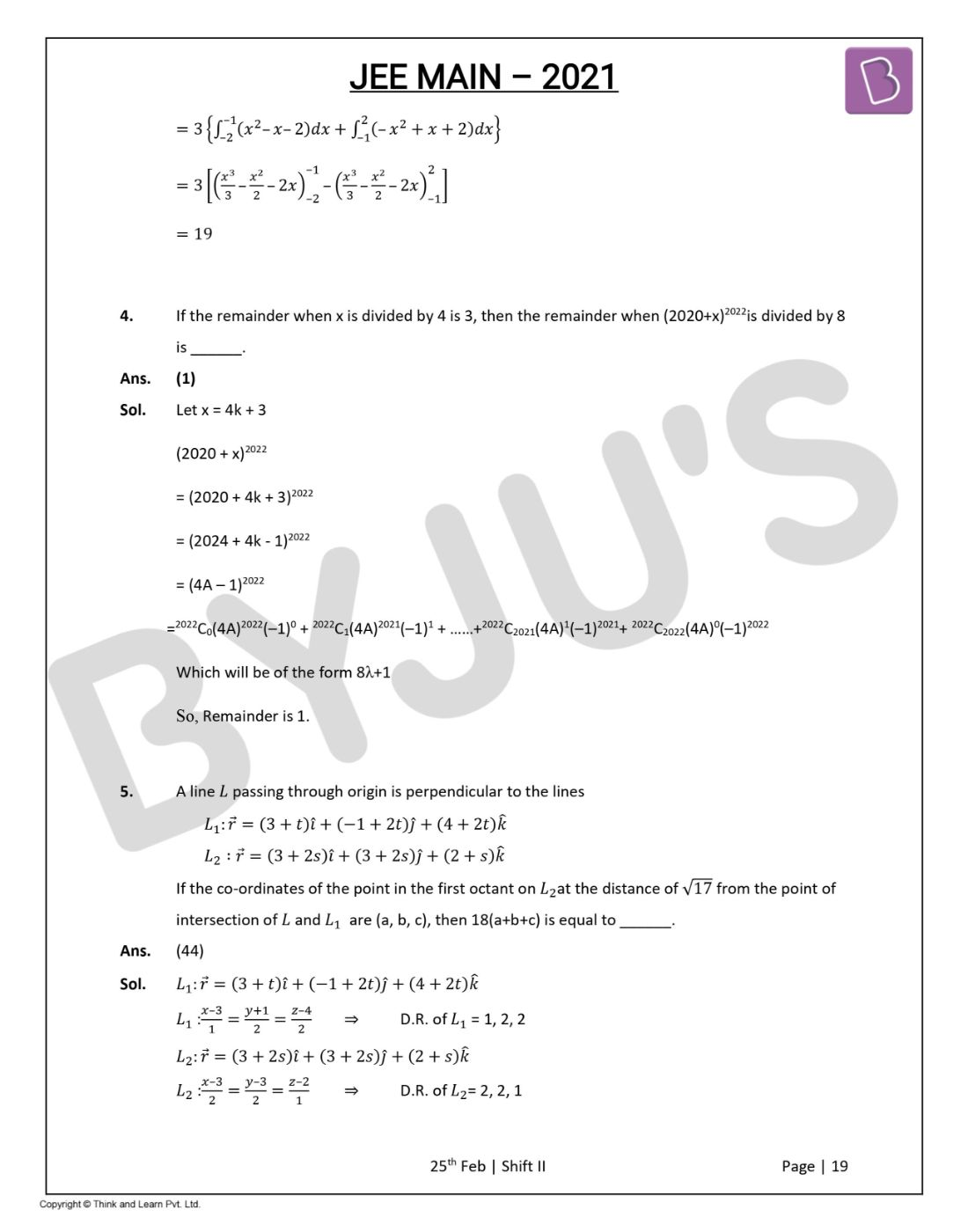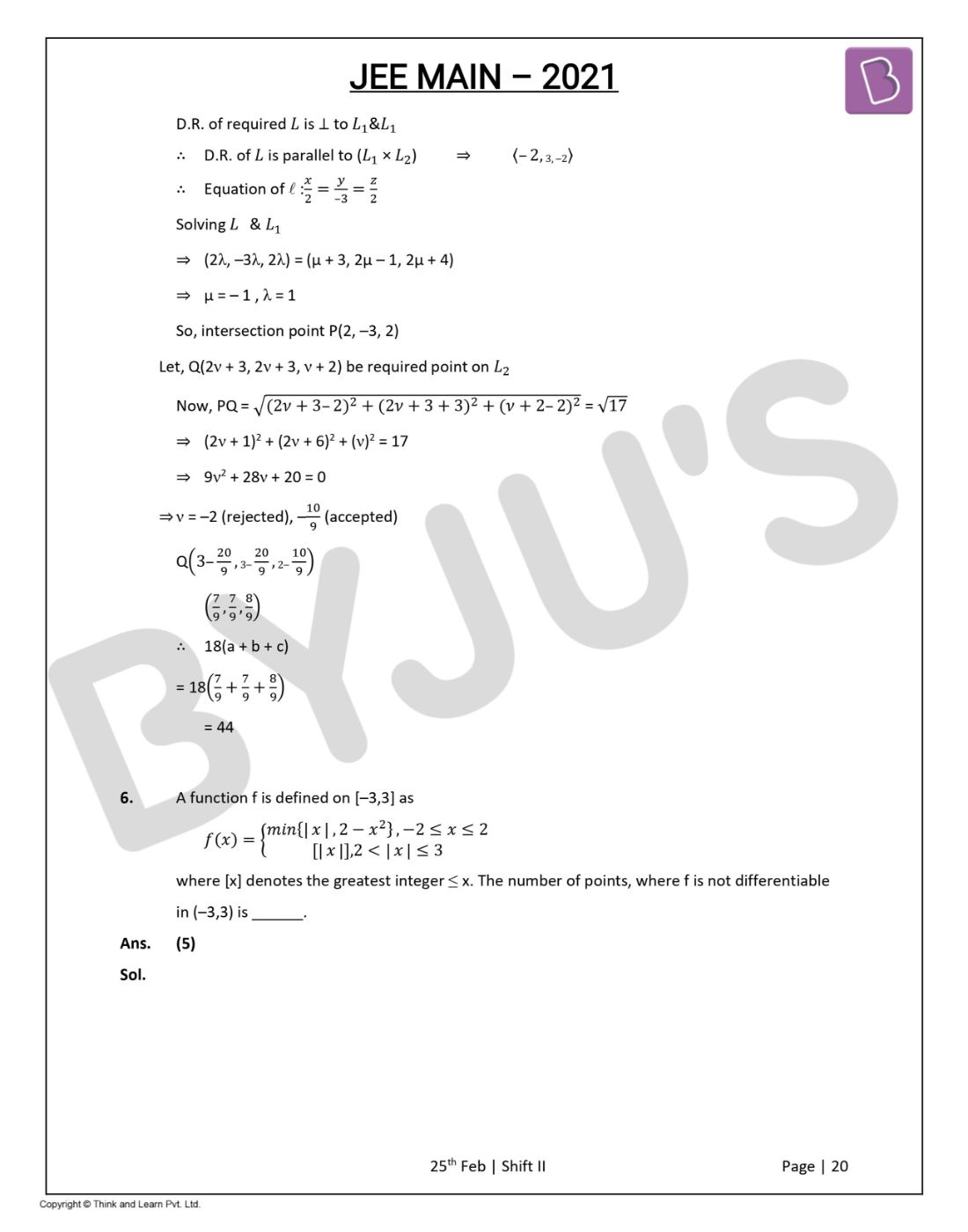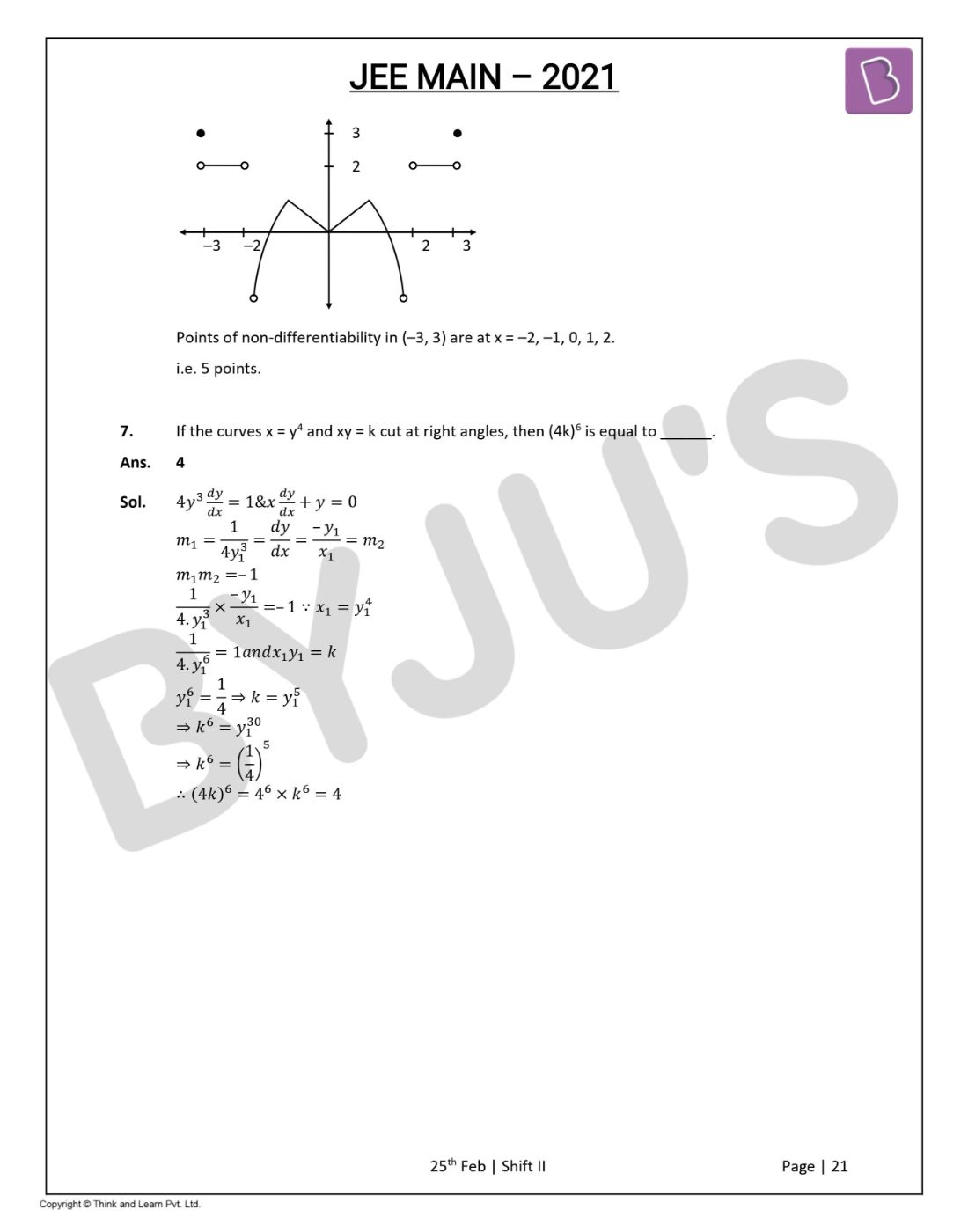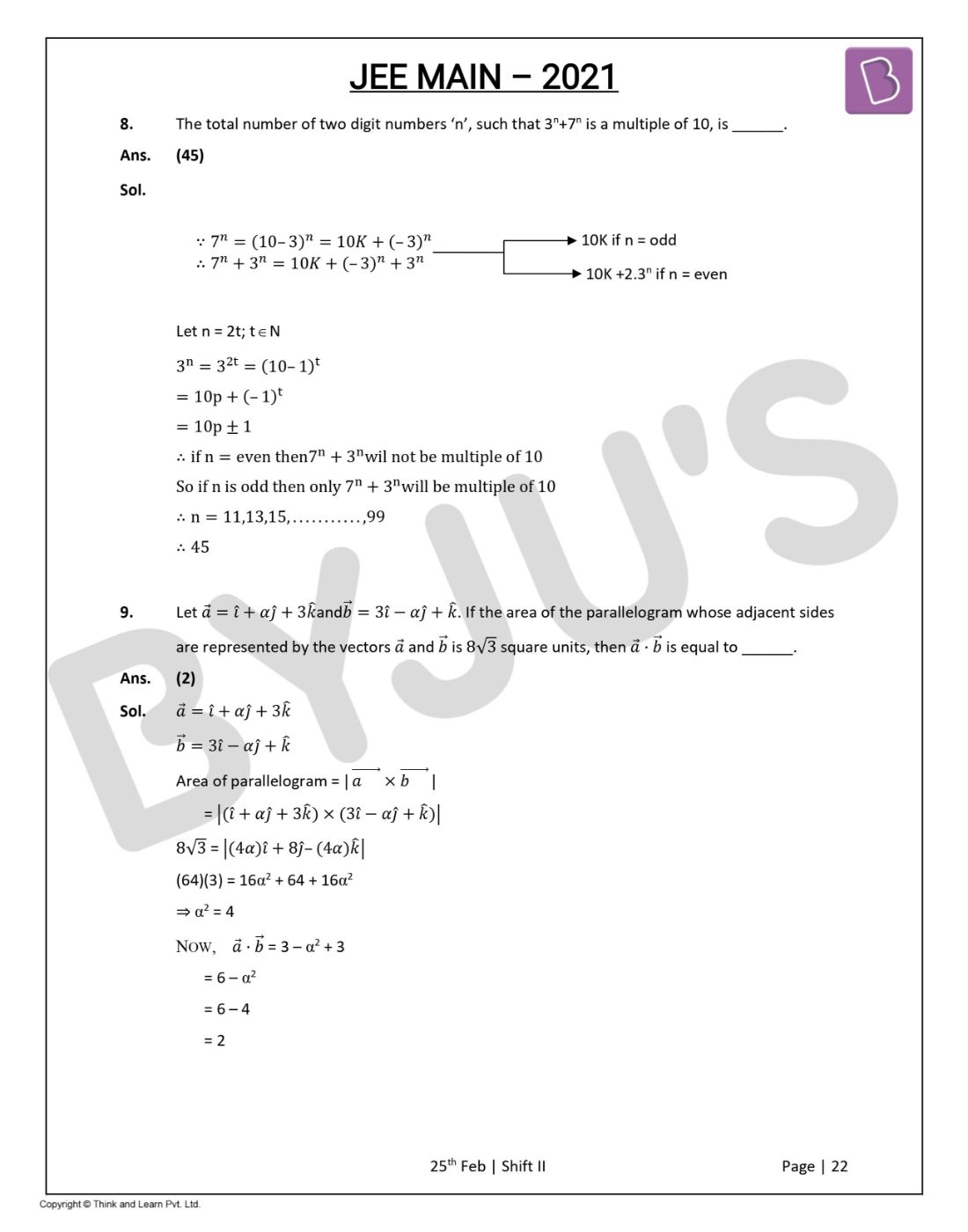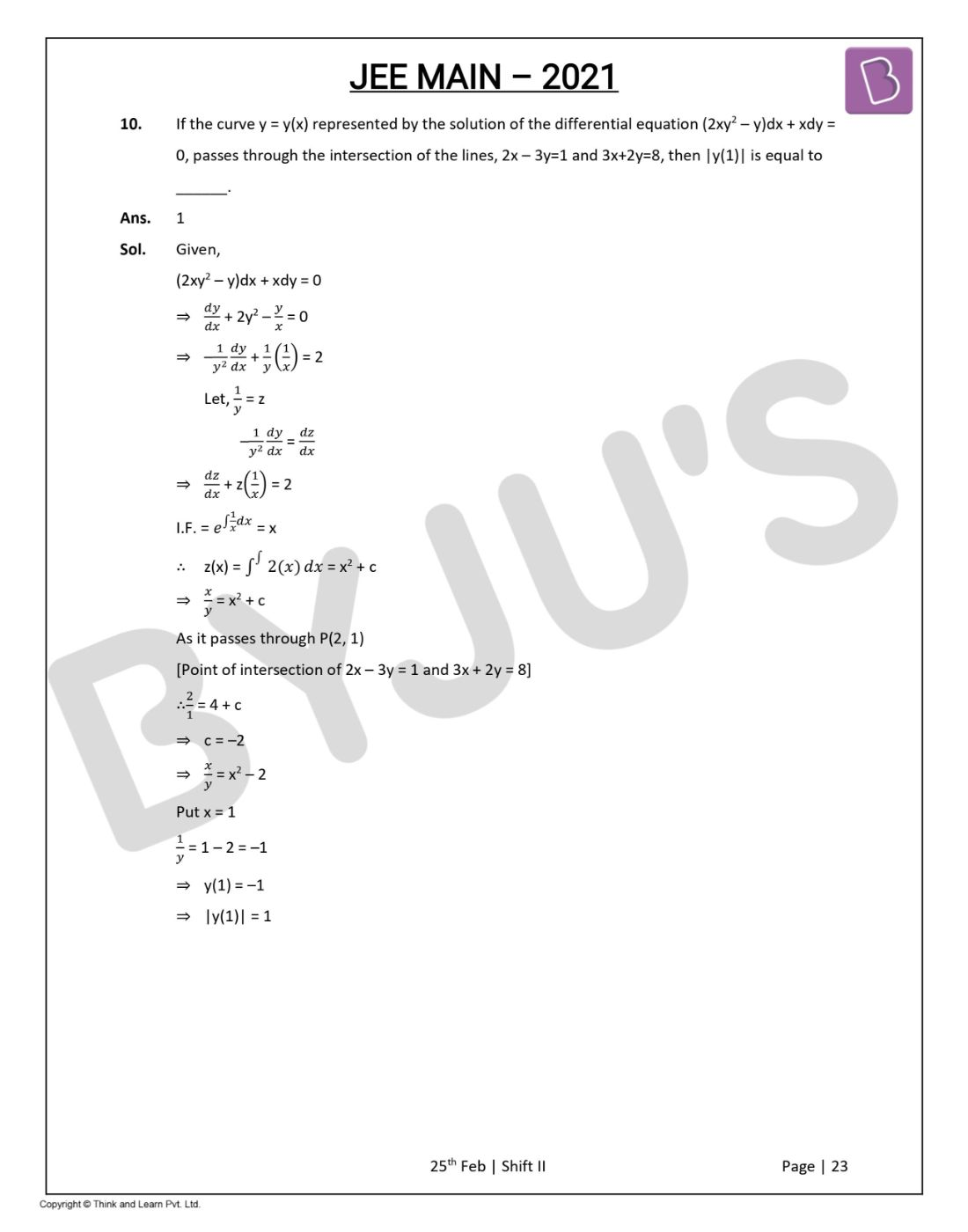Win up to 100% scholarship on Aakash BYJU'S JEE/NEET courses with ABNAT Win up to 100% scholarship on Aakash BYJU'S JEE/NEET courses with ABNAT

# JEE Main 2021 February 25 – Shift 2 Maths Question Paper with Solutions

Here, candidates will find JEE Main 2021 Maths shift 2 question paper with solutions. Candidates who appeared in the JEE Main Maths shift 2 exam 2021 can also watch the below video to check the correct answers and analyze their performance. The candidates will also get detailed solutions along with answers in the JEE Main 2021 February 25 Shift 2 Maths question paper PDFs.

JEE Main 2021 25th February Shift 2 Maths Question Paper

SECTION A

Question 1: A plane passes through the points A(1,2,3), B(2,3,1) and C(2,4,2). If O is the origin and P is (2,–1,1), then the projection of vector(OP) on this plane is of length:

a. √(2/5)
b. √(2/3)
c. √(2/11)
d. √(2/7)

A(1,2,3),B(2,3,1),C(2,4,2),O(0,0,0)

Equation of plane passing through A, B, C will be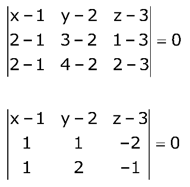(x – 1)(–1 + 4) – (y – 2)(–1 + 2) + (z – 3)(2 – 1) = 0

(x – 1)(3) – (y – 2)(1) + (z – 3)(1) = 0

3x – 3 – y + 2 + z – 3 = 0

3x – y + z – 4 = 0, is the required plane equation

Now, given O(0,0,0) & P(2,–1,1)

Plane is 3x – y + z – 4 = 0

Let O’ & P’ be the foot of perpendiculars.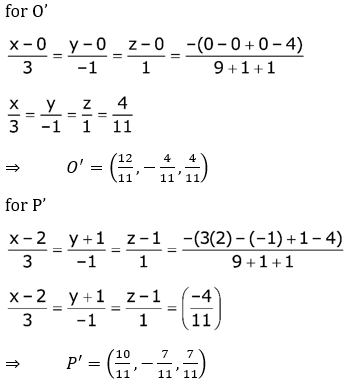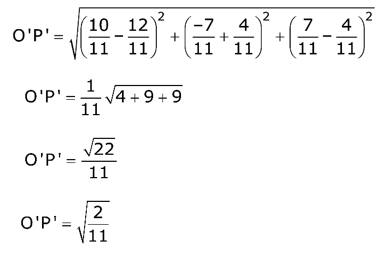Question 2: The contrapositive of the statement “If you will work, you will earn money” is:

a. If you will not earn money, you will not work
b. You will earn money, if you will not work
c. If you will earn money, you will work
d. To earn money, you need to work

Contrapositive of p → q is ~q →~p

p: you will work

q: you will earn money

~q: you will not earn money

~p: you will not work

~q →~p: if you will not earn money, you will not work

Question 3: If α, β∈ R are such that 1 – 2i (here i2 = –1) is a root of z2 + αz + β = 0, then (α – β) is equal to:

a. 7
b. -3
c. 3
d. -7

1-2i is a root of z2 + αz + β = 0.

(1-2i) 2 + α(1-2i)+β=0

⇒1-4-4i+α-2iα+β=0

⇒(α+β-3)-i(4+2α)=0

⇒α+β-3=0 & 4+2α=0

So, α=-2, β=5

Therefore, α-β=-7

Question 4: If

$$\begin{array}{l}\int_{\pi/4}^{\pi/2}cot^nx dx\end{array}$$
, then

a. 1/(I2 + I4 ) , 1/(I3 + I5 ), 1/(I4 + I6) are in G.P.
b. 1/(I2 + I4 ) , 1/(I3 + I5 ), 1/(I4 + I6) are in A.P.
c. I2 + I4 , I3 + I5 , I4 + I6) are in A.P.
d. I2 + I4 , I3 + I5 , I4 + I6) are in G.P.

$$\begin{array}{l}I_{n+2}+I_n = \int_{\pi/4}^{\pi/2}cot^nx . cosec^2x dx = \(\begin{array}{l}\frac{-(cotx)^{n+1}}{n+1}]_{\pi/4}^{\pi/2}\end{array}$$

In+2 + In = 1/(n+1)

I2 + I4 =1/3, I3 + I5 =1/4 and I4 + I6 =1/5

So, 1/(I2 + I4 ) , 1/(I3 + I5 ) and 1/(I4 + I6) are in A.P.

Question 5: If for the matrix,

$$\begin{array}{l}A = \begin{bmatrix} 1 & -\alpha \\ \alpha & \beta \end{bmatrix}\end{array}$$
AAT = I2, then the value of α4 + β4 is:

a. 1
b. 3
c. 2
d. 4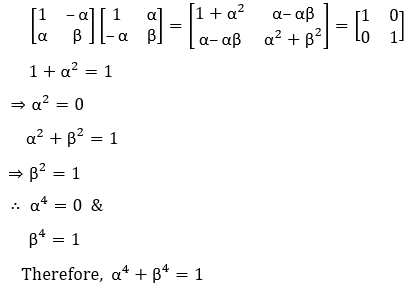Question 6: Let x denote the total number of one-one functions from a set A with 3 elements to a set B with 5 elements and y denote the total number of one-one functions from the set A to the set A × B. Then:

a. y = 273x
b. 2y = 91x
c. y = 91x
d. 2y = 273x

Number of elements in A = 3

Number of elements in B = 5

Number of elements in A × B = 15

Number of one-one function is x = 5 × 4 × 3

⇒x = 60

Number of one-one function is y = 15 × 14 × 13

⇒y = 15 × 4 × 14/4 × 13

⇒y = 60 × 7/2 × 13

⇒2y = (13)(7x)

⇒2y = 91x

Question 7: If the curve x2 + 2y2 = 2 intersects the line x + y = 1 at two points P and Q, then the angle subtended by the line segment PQ at the origin is:

a. ??/2 + tan–1(1/4)
b. ??/2 – ??????–1(1/4)
c. ??/2 + ??????–1(1/3)
d. ??/2 – ??????–1(1/3)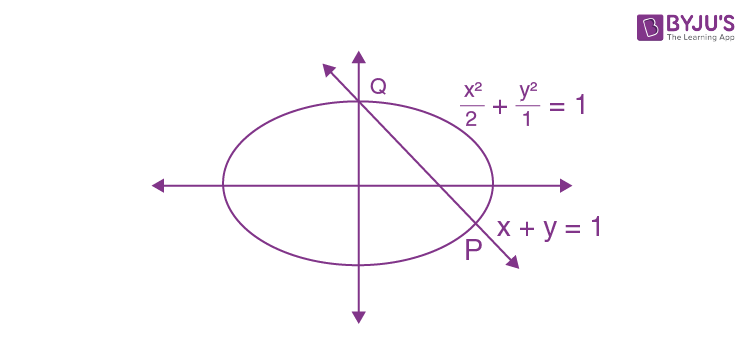Using homogenization

x2 + 2y2 = 2(1) 2

⇒x2 + 2y2 = 2(x + y) 2

⇒x2 + 2y2 = 2x2 + 2y2 + 4xy

⇒x2 + 4xy = 0

for ax2 + 2hxy + by2 = 0, obtuse angle between lines θ is

tan θ = ±(2√(h2–ab))/(a+b)

⇒tan θ = ±4

⇒tan θ = –4

cot θ = -1/4

θ = cot–1(–1/4)

θ = π – cot–1 (1/4)

θ = π – (π/2– tan–1(1/4) )

θ = π/2 + tan-1(1/4)

Question 8: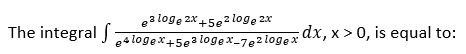a. loge|x2 + 5x – 7| + c
b. (1/4) loge |x2 + 5x – 7| + c
c. 4 loge|x2 + 5x – 7| + c
d. loge √(x2 + 5x – 7) + cQuestion 9: A hyperbola passes through the foci of the ellipse x2/25 + y2/16 = 1 and its transverse and conjugate axes coincide with major and minor axes of the ellipse, respectively. If the product of their eccentricities is one, then the equation of the hyperbola is:

a. x2/9 – y2/4 = 1
b. x2/9 – y2/16 = 1
c. x2 – y2 = 9
d. x2/9 – y2/25 = 1

For ellipse, e1 = √(1–16/25)=3/5

Foci = (±3,0)

Let equation of hyperbola be x2/A2 – y2/B2 = 1, passes through (±3, 0)

A2 =9, A=3, e2=5/3

e22 = 1 + B2/A2

25/9 = 1 + B2/9 ⇒B2 = 16

x2/9 – y2/16 = 1

Question 10:

$$\begin{array}{l}\lim_{n \rightarrow \infty}[\frac{1}{n} + \frac{n}{(n+1)^2} + \frac{n}{(n+2)^2} +……+ \frac{n}{(2n-1)^2}]\end{array}$$
is equal to:

a. 1
b. 1/3
c. 1/2
d. 1/4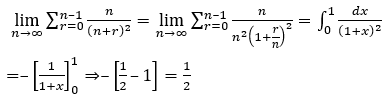Question 11: In a group of 400 people, 160 are smokers and non-vegetarian; 100 are smokers and vegetarian and the remaining 140 are non-smokers and vegetarian. Their chances of getting a particular chest disorder are 35%, 20% and 10% respectively. A person is chosen from the group at random and is found to be suffering from chest disorder. The probability that the selected person is a smoker and non-vegetarian is:

a. 7/45
b. 8/45
c. 14/45
d. 28/45

Based on Bayes’ theorem

Probability = ((160/400×35/100))/((160/400×35/100)+(100/400×20/100)+(140/400×10/100) )

= 5600/9000

= 28/45

Question 12: The following system of linear equations

2x + 3y + 2z = 9

3x + 2y + 2z = 9

x – y + 4z = 8

a. does not have any solution
b. has a unique solution
c. has a solution (α, β, γ) satisfying α + β2 + γ3 = 12
d. has infinitely many solutions

Determinant of given system = -20 ≠ 0

It has unique solution.

Question 13: The minimum value of

$$\begin{array}{l}f(x) = a^{a^{x}} + a^{1-a^{x}}\end{array}$$
where a, x ∈ R and a > 0, is equal to:

a. a + 1/a
b. a + 1
c. 2a
d. 2√a

Using AM ≥ GM inequality, we get

$$\begin{array}{l}\frac{a^{ax}+\frac{a}{a^{ax}}}{2}\geq \left ( a^{ax}.\frac{a}{a^{ax}} \right )^{\frac{1}{2}} \Rightarrow a^{ax} + a^{1-ax}\geq 2\sqrt{a}\end{array}$$

Question 14: A function f(x) is given by f(x) = 5x/(5x+5), then the sum of the series f(1/20) + f(2/20) + f(3/20) + …… + f(39/20) is equal to:

a. 19/2
b. 49/2
c. 39/2
d. 29/2

f(x) = 5x/(5 x + 5)….(i)

⇒ f(2–x) = 52–x/(52–x +5)

⇒f(2–x) = 5/(5x+5)……(ii)

f(x)+f(2–x)=1

f(1/20)+f(39/20)=1

f(2/20)+f(38/20)=1

……

…..

f(19/20) + f(21/20)=1

and f(20/20) = f(1) = 1/2

Therefore, f(1/20) + f(2/20) + f(3/20) + …… + f(39/20) = 19 + 1/2 = 39/2

Question 15: Let α and β be the roots of x2 – 6x – 2 = 0. If an = αn – βn for n ≥ 1, then the value of (a10–2a8)/(3a9 ) is:

a. 4
b. 1
c. 2
d. 3

α and β be the roots of x2–6x–2=0.

So,

α2–6α–2=0⇒α2–2=6α

β2–6β–2=0⇒β2–2=6β

Now,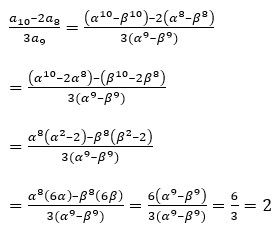Question 16: Let A be a 3 × 3 matrix with det(A) = 4. Let Ri denote the ith row of A. If a matrix B is obtained by performing the operation R2→ 2R2 + 5R3 on 2A, then det(B) is equal to:

a. 64
b. 16
c. 80
d. 128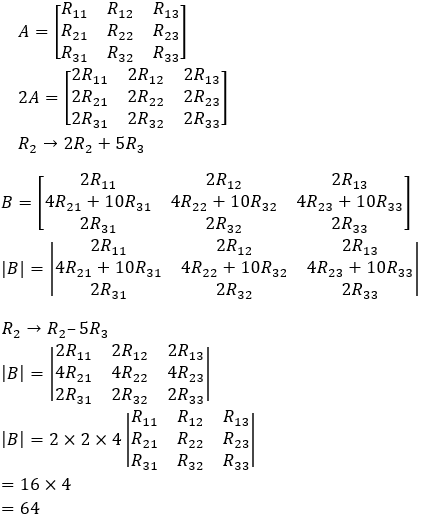Question 17: The shortest distance between the line x – y = 1 and the curve x2 = 2y is:

a. 1/2
b. 0
c. 1/(2√2)
d. 1/√2

Shortest distance must be along common normal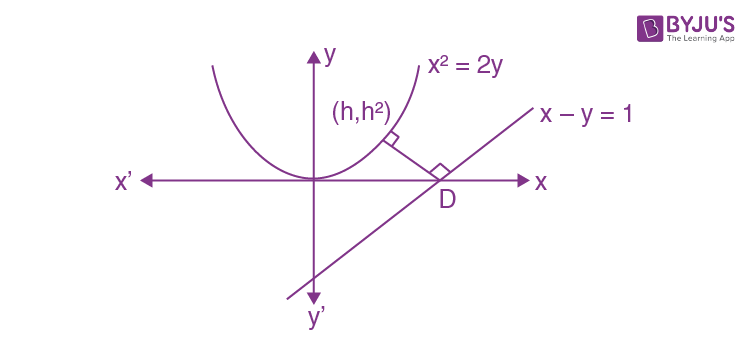m1 (slope of line x–y = 1) = 1 ⇒slope of perpendicular line =–1

m2 = 2x/2 = x ⇒ m2 = h ⇒slope of normal = –1/h

–1/h=–1 ⇒h=1

So, the point is (1,1/2)

Therefore, Shortest distance (D) =|(1–1/2–1)/√(1+1)|=1/(2√2)

Question 18: Let A be a set of all 4-digit natural numbers whose exactly one digit is 7. Then the probability that a randomly chosen element of A leaves remainder 2 when divided by 5 is:

a. 1/5
b. 2/9
c. 97/297
d. 122/297

Total cases

= 4C1 × 9 × 9 × 9 – 3C1 × 9 × 9

(as 4 digit number having 0 at thousands place have to be excluded)

For a number to have a remainder 2 when divided by 5 it’s unit digit should be 2 or 7

Case 1: when unit digit is 2

Number of four-digit number = 3C1×9×9 – 2C1×9

Case 2: when unit digit is 7

Number of four digits number = 8 × 9 × 9

So total number favorable cases = 3×92 – 2×9 + 8×92

Required Probability = ((3×9×9)–(2×9)+(8×9×9))/((4×93) – (3×92))

= 97/297

Question 19: cosec[2 cot–1( 5) + cos–1(4/5) ] is equal to:

a. 75/56
b. 65/56
c. 56/33
d. 65/33

cosec[2 cot–1( 5) + cos–1(4/5) ]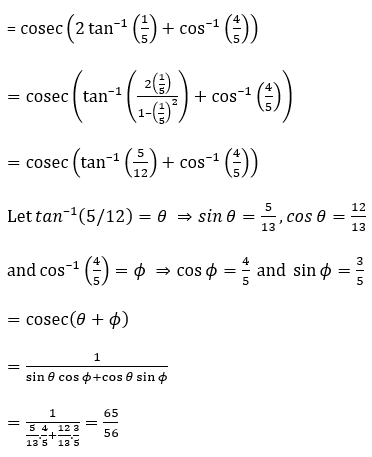Question 20: If 0 < x, y < π and cos x + cos y – cos (x + y) = 3/2, then sin x + cos y is equal to:

a. (1+√3)/2
b. (1–√3)/2
c. √3/2
d. 1/2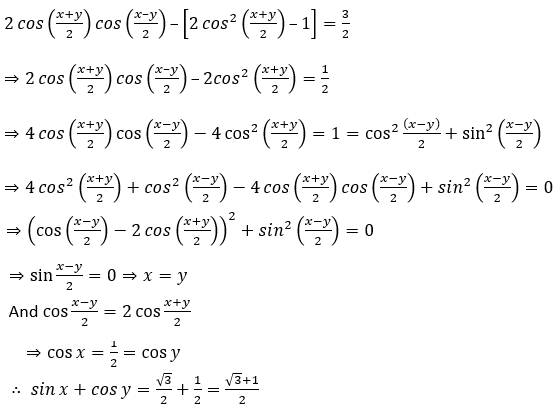Section B

Question 1: If

$$\begin{array}{l}\lim_{x \rightarrow 0} \frac{ax-(e^{4x}-1)}{ax(e^{4x}-1)}\end{array}$$
exists and is equal to b, then the value of a – 2b is ______.

$$\begin{array}{l}\lim_{x \rightarrow 0} \frac{ax-(e^{4x}-1)}{ax(e^{4x}-1)}\end{array}$$

Applying L’Hospital Rule

$$\begin{array}{l}\lim_{x \rightarrow 0} \frac{a-4e^{4x}}{a(e^{4x}-1)+ax(4e^{4x})}\end{array}$$
So for limit to exist,a=4

Applying L’Hospital Rule

$$\begin{array}{l}\lim_{x \rightarrow 0} \frac{-16e^{4x}}{a(4e^{4x}) +a(4e^{4x})+ax(16e^{4x})}\end{array}$$

(-16)/(4a+4a)=(–16)/32=–1/2=b

a-2b = 4–2((–1)/2) = 4+1 = 5

Question 2: A line is a common tangent to the circle (x – 3)2 + y2 = 9 and the parabola y2 = 4x. If the two points of contact (a, b) and (c, d) are distinct and lie in the first quadrant, then 2(a+c) is equal to ______.

Sol. Circle: (x – 3)2+ y2= 9

Parabola: y2 = 4x

Let common tangent equation be y = mx + a/m

⇒y = mx + 1/m

⇒m2x – my + 1 = 0

The above line is also tangent to circle

(x – 3)2 + y2= 9

Therefore, the perpendicular from (3, 0) to line = 3

⇒|(3m2 – 0 + 1)/√(m2+m4 )| = 3

⇒(3m2 + 1)2 = 9(m2 + m4)

⇒m = ±1/√3

Tangent is

y = 1/√3x + √3 (it will be used)

=> m = 1/√3

or y = –1/√3x – √3 (rejected)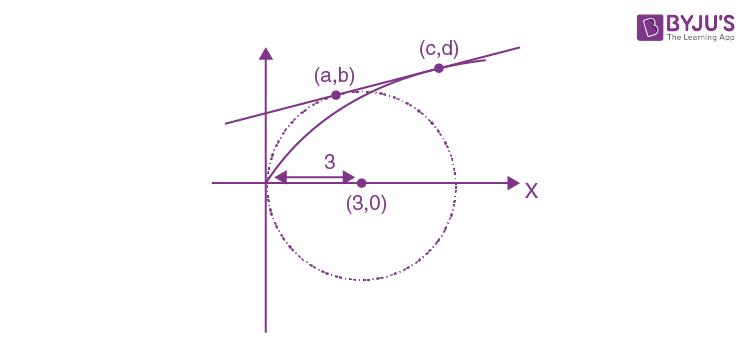For Parabola, point of contact is (a/m2 , 2a/m)= (3, 2√3) = (c, d)

Solving Circle (x – 3)2 + y2 = 9 & line equation y = (1/√3) x + √3

(x – 3)2 + ((1/√3) x + √3) 2 = 9

⇒x2 + 9 – 6x + (1/3)x2 + 3 + 2x = 9

⇒(4/3)x2 – 4x + 3 = 0

⇒x = 3/2=a

∴ 2(a + c) = 2(3/2+3)= 9

Question 3: The value of

$$\begin{array}{l}\int_{-2}^2|3x^2-3x-6|dx\end{array}$$
is ______.

x2-x-2=(x–2)(x+1)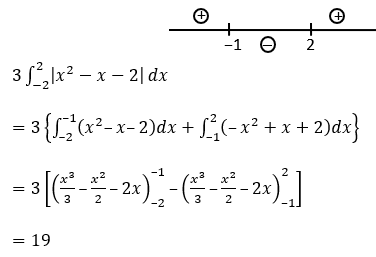Question 4: If the remainder when x is divided by 4 is 3, then the remainder when (2020+x)2022 is divided by 8 is ______.

Let x = 4k + 3

(2020 + x)2022

= (2020 + 4k + 3)2022

= (2024 + 4k – 1)2022

= (4A – 1)2022

=2022C0(4A)2022(–1)0 + 2022C1(4A)2021(–1)1 + ……+2022C2021(4A)1(–1)2021+ 2022C2022(4A)0(–1)2022

Which will be of the form 8λ+1

So, Remainder is 1.

Question 5: A line L passing through origin is perpendicular to the lines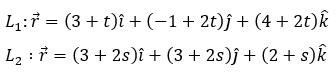If the co-ordinates of the point in the first octant on L2 at the distance of √17 from the point of intersection of L and L1 are (a, b, c), then 18(a+b+c) is equal to ______.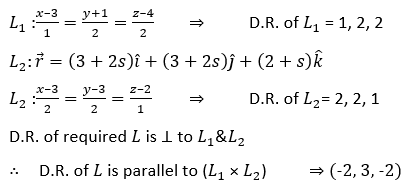D.R. of L is parallel to (L1 × L2) ⇒ (-2, 3, -2)

Equation of l : x/2 = y/(–3) = z/2

Solving L & L1

(2λ, –3λ, 2λ) = (µ + 3, 2µ – 1, 2µ + 4)

µ = – 1 , λ = 1

So, intersection point P(2, –3, 2)

Let, Q(2ν + 3, 2ν + 3, ν + 2) be required point on L2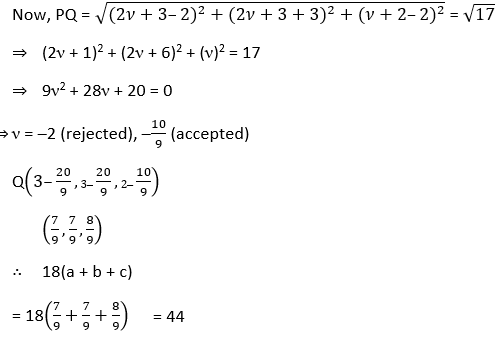Question 6: A function f is defined on [–3,3] as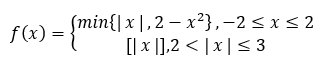where [x] denotes the greatest integer ≤ x. The number of points, where f is not differentiable in (–3,3) is ______.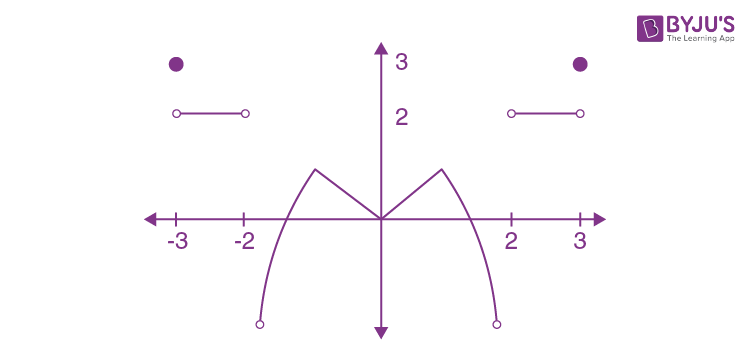Points of non-differentiability in (–3, 3) are at x = –2, –1, 0, 1, 2.

i.e. 5 points.

Question 7: If the curves x = y4 and xy = k cut at right angles, then (4k)6 is equal to ______.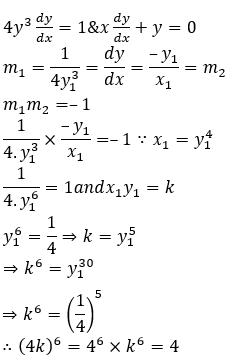Question 8: The total number of two digit numbers ‘n’, such that 3n+7n is a multiple of 10, is ______.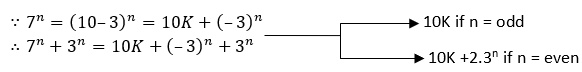Let n = 2t; t ∈ N

3n = 32t = (10 – 1)t

=10p + (–1)t

= 10p ± 1

If n = even then 7n + 3n will not be multiple of 10

So, if n is odd then only 7n + 3n will be a multiple of 10

Therefore, n = 11,13,15,………..,99

Question 9: Let

$$\begin{array}{l}\vec{a} = \hat{i} +\alpha \hat{j} + 3\hat{k} \: and \: \vec{b} = 3\hat{i} -\alpha \hat{j} + \hat{k}\end{array}$$
. If the area of the parallelogram whose adjacent sides are represented by the vectors
$$\begin{array}{l}\vec{a}\end{array}$$
and
$$\begin{array}{l}\vec{a}\end{array}$$
is 8√3 square units, then
$$\begin{array}{l}\vec{a} . \vec{b}\end{array}$$
is equal to ______
.

Area of parallelogram =

$$\begin{array}{l}|\vec{a} \times \vec{b}|\end{array}$$

=

$$\begin{array}{l}|(\hat{i} + \alpha \hat{j} + 3 \hat{k}) \times (3\hat{i} – \alpha \hat{j} + \hat{k})|\end{array}$$

(64)(3) = 16α2 + 64 + 16α2

⇒ α2 = 4

Now,

$$\begin{array}{l}\vec{a}.\vec{b}\end{array}$$
= 3 – α2 + 3

= 6 – α2

= 6 – 4

= 2

Question 10: If the curve y = y(x) represented by the solution of the differential equation (2xy2 – y)dx + xdy = 0, passes through the intersection of the lines, 2x – 3y = 1 and 3x + 2y = 8, then |y(1)| is equal to ______.

Given,

(2xy2 – y)dx + xdy = 0

⇒ dy/dx + 2y2 – y/x = 0

⇒ (–1/y2) . dy/dx + (1/y) (1/x) = 2

Let, 1/y = z

(–1/y2) dy/dx = dz/dx

⇒dz/dx + z(1/x) = 2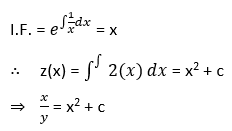As it passes through P(2, 1)

[Point of intersection of 2x – 3y = 1 and 3x + 2y = 8]

Therefore, 2/1 = 4 + c

⇒c = –2

⇒x/y = x2 – 2

Put x = 1

1/y = 1 – 2 = –1

⇒y(1) = –1

⇒|y(1)| = 1

#### Video Solutions- February 2021 Question Papers